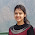### Wire Behavior at High Frequencies

In Ohm's circuit law
V = I*R
Voltage is assumed to be constant.
But at very high frequencies voltage vary due to propagation effect.
Now the question is whether Ohm's law valid at high frequencies or not?

Ohm's law for lumped elements drives from the more general Ohm's law definition of
J = sigma*E

Where
J is the current density at a given location in a resistive material.

E is the electric Field at that location.
sigma is conductivity that is material dependent parameter.

Thus Ohm's law is valid for higher frequencies.

σ = f(position, frequency). On lower frequencies we can neglect propagation effect (circuit is small compared to propagation wave) and we have only σ = f(frequency).

Now

Wave length of electromagnetic wave at 1 Terahertz frequency:

L (Wave length) = C/f
L         = (3x10^8)/ 10^12
L         = 0.3 cm

So at higher frequencies wavelength becomes small (L = 0.3 cm) and the fields, voltages and currents along wire vary with minima and maxima at each multiple of L/2 (= 0.15 cm). So the impedance will be different at each point.

It is assumed that the wire length smaller than 1/10th of the wave length (< 0.03 cm for terahertz frequencies) result in lumped element behavior. However, bare in mind that the speed of electricity in semiconductor or copper is lower than the speed of light. So, we will have to adjust the values accordingly.

This is why the CMOS works on high frequencies (few GHz).
Let a CMOS circuit is working on 3 GHz frequency.
Wave length of waves at 3 GHz frequency will be:

L (Wave length) = C/f
L         = (3x10^8)/(3x10^9)
L         = 0.1 m

Wave length (L) will be lower than 0.1 meter in CMOS because of semiconductor. So, wire lower than 0.01 meter (= 1 cm) length (smaller than 1/10th of the wave length) will result in lumped element behavior.
It is all about size, size of the circuit and size of waves. Make the circuits small enough to work on high frequencies, otherwise you will have to consider it as a transmission line.

At higher frequencies transfer of energy is based on charging and discharging of electromagnetic waves and storage elements are capacitance and inductive. Thus for the signal that is propagating it seems like a series of C-L-R.
Wire circuit at High Frequencies

The same thing happens with long power transmission lines even at 50 Hz frequencies. A long power line (let say 200 km long) shows distributed nature.

#### 1 comment:

1.this information is very useful..nice efforts done